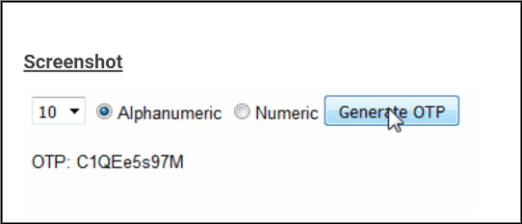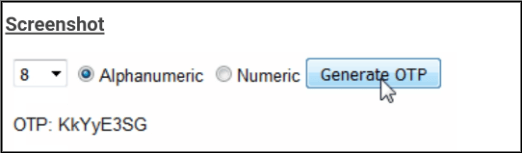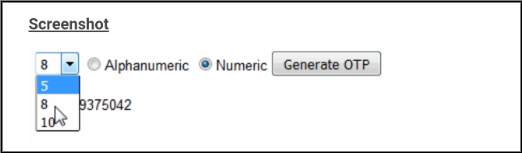# How to Generate One Time Password(OTP) in Visual basic (VB.net)

How to Generate One Time Password(OTP) using VB.NET. In this article, we will learn How to Generate One Time Password(OTP) in Visual Basic (VB.NET).

## What is OTP?

VB.Net Login Using Access Database ...
VB.Net Login Using Access Database 2021 With Source Code | VB.Net Login Form Example

OTP stands for a one-time password. It is a password that is valid for only one login session or transaction. It can be numeric as well as alphanumerical.OTP (One Time Passwords) are widely used by banks and other firms to validate the Mobile Numbers of their users. OTP’s generally have lengths between 5-10 characters.

The simplest example of an OTP is when you try to connect to a public Wi-Fi network, your mobile device receives an SMS containing a unique password and once you register with the correct password, the device gets connected to the network.

### Visual Basic Code to Generate numeric OTP

Write the below-given code on the button click event to generate the numeric OTP.

```Protected Sub Button1_Click(ByVal sender As Object, ByVal e As System.EventArgs) Handles Button1.Click
GenerateOTP()
End Sub

Private Sub GenerateOTP()
Try
Dim length As Integer = 6
Dim numbers As String = "0123456789"
Dim objrandom As New Random()
Dim strrandom As String = String.Empty
Dim noofnumbers As Integer = length
For i As Integer = 0 To noofnumbers - 1
Dim temp As Integer = objrandom.[Next](0, numbers.Length)
strrandom += temp
Next
lblotp.Text = strrandom
idotp.Visible = True
Catch ex As Exception
End Try
End Sub```

When the Button is clicked, the length and the type values of the Password are fetched from the DropDownList and RadioButtonList. There are three strings,

• First containing Upper Case Alphabets.
• Second, containing Lower Case Alphabets.
• Third one containing the 10 digits.

Then a loop is executed and inside the loop, a random number is used to fetch the character from the combination of Alphabets and Numeric strings or only the Numeric string based on the type selected in the RadioButtonList.

Inside the For Loop, a While loop is used to avoid repetition of the characters.

The generated unique random OTP i.e. One Time Password is displayed in the Label control.

### Generate alphanumeric OTP in VB.NET

Write the below-given code on the button click event to generate the alphanumeric OTP.

```Protected Sub GenerateOTP(sender As Object, e As EventArgs)
Dim alphabets As String = "ABCDEFGHIJKLMNOPQRSTUVWXYZ"
Dim small_alphabets As String = "abcdefghijklmnopqrstuvwxyz"
Dim numbers As String = "1234567890"

Dim characters As String = numbers
If rbType.SelectedItem.Value = "1" Then
characters += Convert.ToString(alphabets & small_alphabets) & numbers
End If
Dim length As Integer = Integer.Parse(ddlLength.SelectedItem.Value)
Dim otp As String = String.Empty
For i As Integer = 0 To length - 1
Dim character As String = String.Empty
Do
Dim index As Integer = New Random().Next(0, characters.Length)
character = characters.ToCharArray()(index).ToString()
Loop While otp.IndexOf(character) <> -1
otp += character
Next
lblOTP.Text = otp
End Sub```

Build the above application and run. To test the working click on generate OTP button.In this way, we learn How to Generate One Time Password(OTP) using VB.NET.